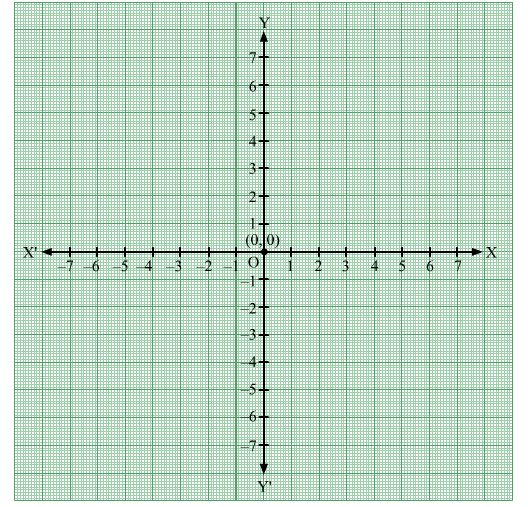# On the plane of a graph paper draw X'OX and YOY' as coordinate axes and plot each of the following points.

Question:

On the plane of a graph paper draw X'OX and YOY' as coordinate axes and plot each of the following points.
(i) A(5, 3)
(ii) B(6, 2)
(iii) C(–5, 3)
(iv) D(4, –6)
(v) E(–3, –2)
(vi) F(–4, 4)
(vii) G(3, –4)
(viii) H(5, 0)
(ix) I(0, 6)
(x) J(–3, 0)
(xi) K(0, –2)
(xii) O(0, 0)

Solution:

(i)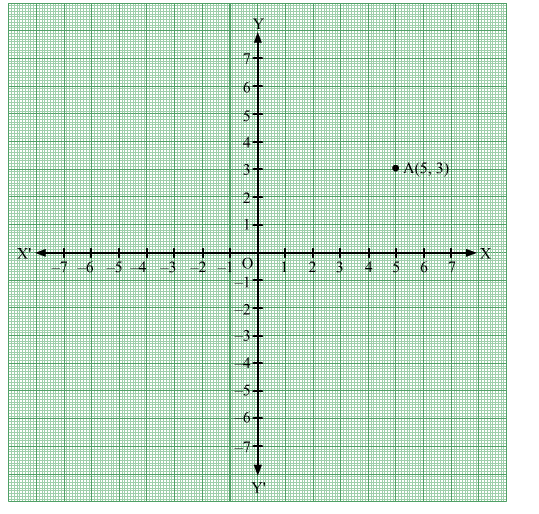(ii)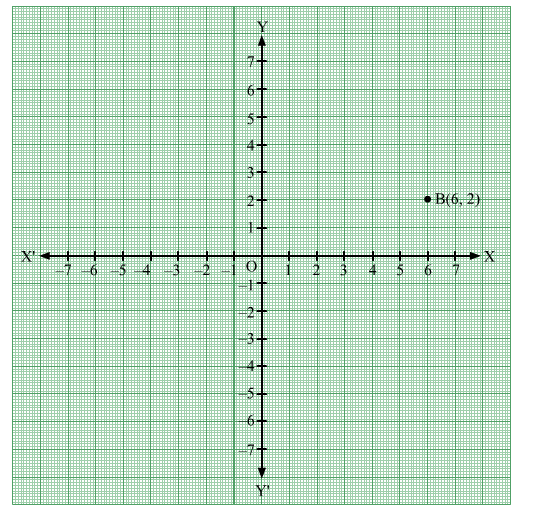(iii)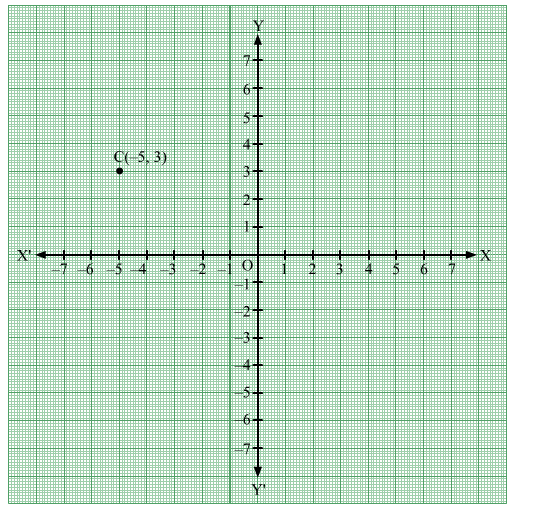(iv)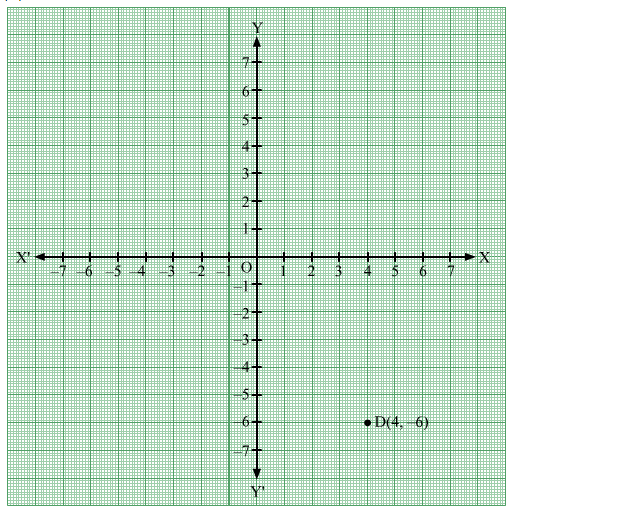(v)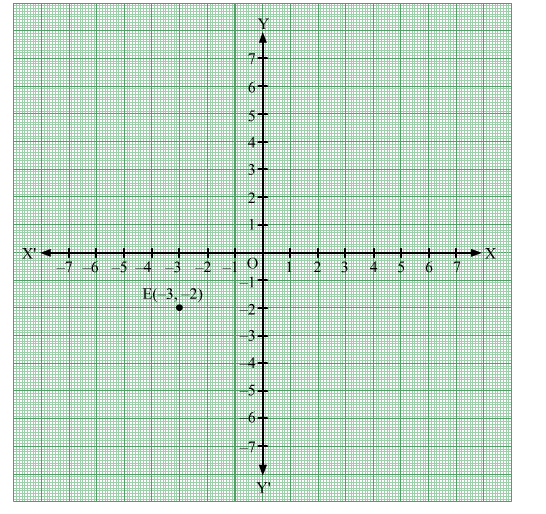(vi)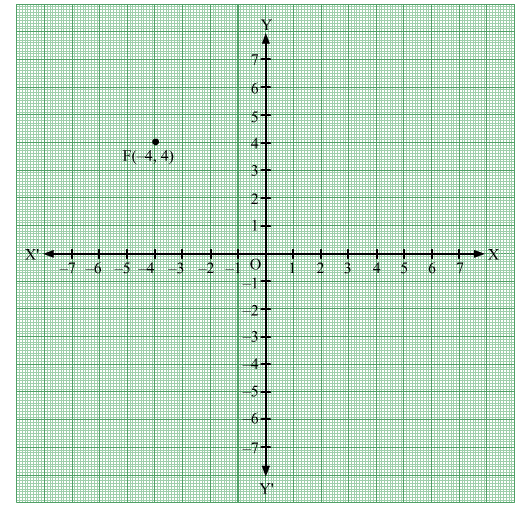(vii)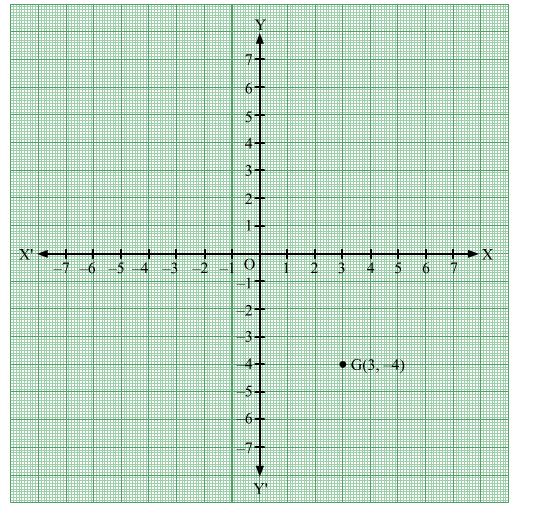(viii)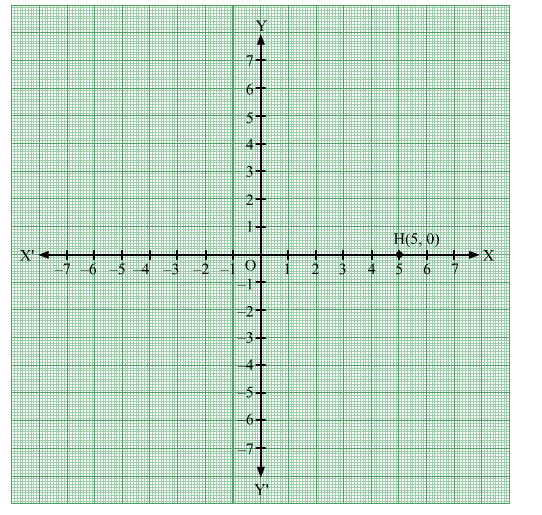(ix)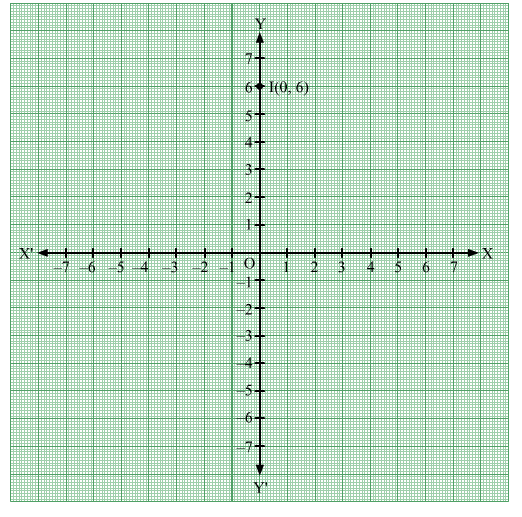(x)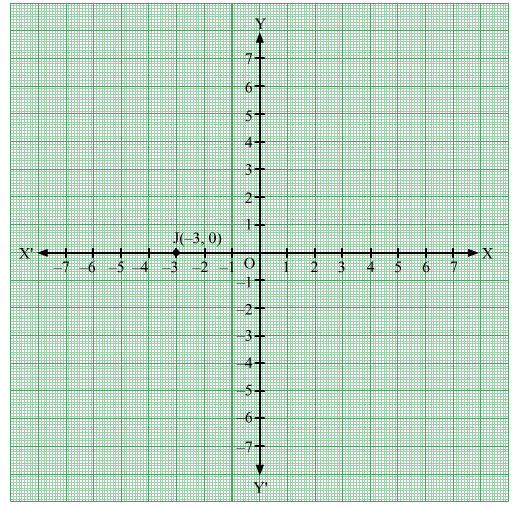(xi)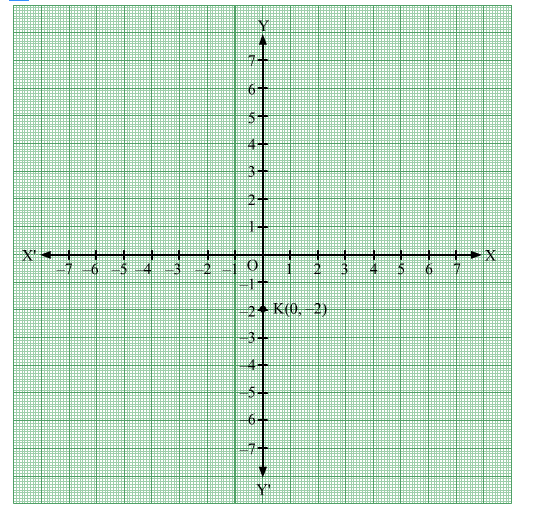(xii)